Reach Us+44 1704 335730
Simple Technique of Projected Lagrange for a Class of Multi-Stage Stochastic Nonlinear Programs | OMICS International
Global Journal of Technology and Optimization
All submissions of the EM system will be redirected to Online Manuscript Submission System. Authors are requested to submit articles directly to Online Manuscript Submission System of respective journal.

# Simple Technique of Projected Lagrange for a Class of Multi-Stage Stochastic Nonlinear Programs

Ihda Hasbiyati1*, Saib Suwilo2, Opim Salim2 and Tulus2

1Departement of Mathematics University of Ria, Indonesia and Graduate School of Departement of Mathematics University of North Sumatra, Indonesia

2Departement of Mathematics University of North Sumatra Indonesia

Corresponding Author:
Ihda Hasbiyati
Departement of Mathematics University of Ria
Indonesia and Graduate School of Departement of Mathematics University of North Sumatra, Indonesia
Tel: 62-81365767274
E-mail: [email protected]

Received Date: March 16, 2015; Accepted Date: March 23, 2015; Published Date: April 07, 2015

Citation: Hasbiyati I, Suwilo S, Salim O, Tulus (2015) Simple Techniq of Projected Lagrangefora Class of Multi-Stage Stochastic Nonlinear Programs. Global J Technol Optim 6:179. doi: 10.4172/2229-8711.1000179

Copyright: © 2015 Hasbiyati I, et al. This is an open-access article distributed under the terms of the Creative Commons Attribution License, which permits unrestricted use, distribution, and reproduction in any medium, provided the original author and source are credited.

Visit for more related articles at Global Journal of Technology and Optimization

#### Abstract

This paper presents a techniq for solving multi-stage stochastic nonlinear programs. The techniq is based on projected lagrange approach which generates the search direction by solving parallelly a set of quadratic programming subproblems with size much less than the original problem at each iteration. Mathamatically, can be pointed out that Lagrange’s projection method can solve problem multi-stage stochastic nonlinear programs.

#### Keywords

Multi-stage stochastic nonlinear programs; Projected langrange; Scenario analysis; Decomposition

#### Introduction

Multi-stage stochastic nonlinear programs emerges in there are many practical situation, as production and manpower planning, portfolio’s selection etc.. Have a lot of research that gets contribution to solve Multi-stage stochastic nonlinear programs amongst those methodics decomposition that is utilized on nonliner’s program also linear [1,2]. Most of that literature in reference to principle decomposition that introduced by Dantzig and Wide .

Decomposition method experience development which is with marks sense L-shape decomposition method that enough effective being utilized to troubleshoot multi-stage stochastic nonlinear programs.

Severally methods the other to troubleshoot multi-stage stochastic nonlinear programs is analyzed among those by Birge . Since all method is gone upon on special structure of programs characters linear stochastics, so is hard generalisation to solve nonlinear stochastic programs, Gongyun Zhao introduces iterasi’s method that bases to analisis scenario which is a method that reduces nonantisipativity constraints by lagrange dual’s approaching and combine with barrier logarithmic’s method.

Hereafter Gongyun Zhao  developing decomposition method which is by use of sequential quadratic method’s programming. Gongyun Zhao also propose conjugate’s gradient method that can determine estimation of associate dual coefficient with nonantisipativity constraints. Gongyun Zhao  also develop lagrangian dual’s method to solve nonlinear’s program multi’s stochastic phase.

Lagrange’s projection algorithm was analyzed by Murtagh and Sander  to troubleshoot sparse nonlinear constraints. Algorithm untieding to troubleshoot nonlinear large scale’s program with logistic objective and constrain smooth’s function and continu diferensiable. Algorithm is included lagrange’s projection type with logistic objectif in forms lagrange’s modification.

Base research already being done researchers former to solve multi-stage stochastic nonlinear program, in this paper, writer propose a method for solve to program multi-stage stochastic nonlinear programs which is by use of lagrange’s projection method. This method is expected gets to give alternative solution to solve multi-stage stochastic nonlinear programs.

#### Materials and Methods

Consider the following multi-stage stochastic program with recourse: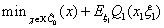(1.1)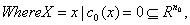the recourse function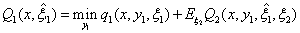Subject to c1 (x, y11 ) = 0 (1.2)

And for t = 2,...,T −1, recursively we have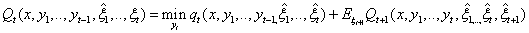(1.3)

Subject to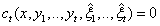(1.4)

QT = 0.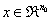is the deterministic vector,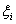is the realization of the random vector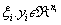is the decision vector in the i-th stage, which is generated recursively by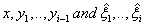hence represents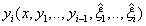actually. cˆ0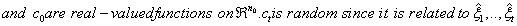For the discrete random vector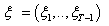if ct has finite realizations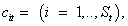then all these cti form the constraint functions on stage t. The details on the formulation of multi-stage stochastic programs can be found, e.g. in Kall and Wallace .

Let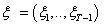and assume that (Ω,θ , P) is the associated probability space. Suppose that we have S scenarios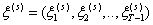which has a fixed and known probability distribution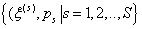Then (1.1)-(1.4) can be reformulated as the following nonlinear programming problem: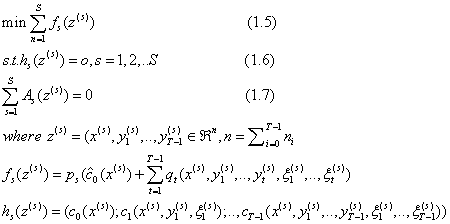Constraints (1.7) are the so-called non-anticipativity constraints, which reflect the fact that scenarios sharing a common history up to any moment of time must have a common decision up to that moment. Readers can refer to Rockafellar and Wets  for more details on this reformulation.

Lagrange’s function in common is deep shaped as follows,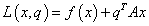For q parameter.

Let say to be given by parameters q and r, therefore form augmented lagrange of equation upon is as follows,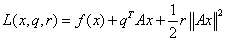Let say to be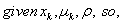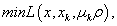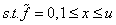To a f = Ax .

Therefore objective function for problem to multi-stage stochastic nonlinear programs is as follows,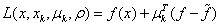#### Result and Discussion

In this paper, Lagrange’s projection method will be utilized for multi-stage stochastic nonlinear programs. The methods based lagrange augmented modified.

Assume that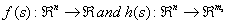are two continu diferensiable functions,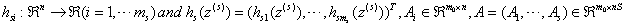is matrix row with full rank and has special structure.

So equation (1.5) – (1.7) are formulated as forms as follows, min f (x)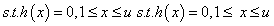A(x) = 0 Where are matrix m x n with m ≤ n .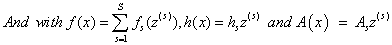That note function of x assumed continu diferensiable.

With Lagrange’s projection method, objektif’s function takings f(x) one equal to form commons of Lagrange augmented’s functions,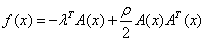Vector λT is lagrange’s coefficient vector and ρ is penalti’s parameter.

Linear Aproksimation from constraints nonlinear is make iterasi along starting point x(k) from iterasi process followings;

A(x(k +1)) = A(x(k)) + h(k)(x(k +1) − x(k))

So algorithm that presented to solve subproblem constrain’s line linear with function objective is modify lagrange augmented and linear aproksimation f(x) on the x(k) are as follows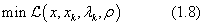s.tA = 0,1≤ x ≤ u

Where is function objective is modify lagrange augmented and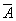is aproksimasi f (x) on the x(k) and,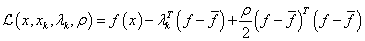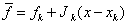Where fk and Jk is cconstraints vector and jacobi’s matrix that evaluated with x(k) .

Definition 1. To ρ = 0 ,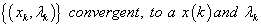one that constitute Lagrange’s solution and coefficient that corespondence to a subproblem.

Definition following to give convex requisite to form lagrange’s modification.

Definition 2.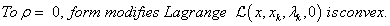Solving problem multi-stage stochastic nonlinear programs by use of lagrange’s projection method depends on penalti’s parameter ρ . If ρ too large therefore will be hard to find solution. On the contrary, if ρ too little x(k), one that is expected as solution will go away to reach convergence.

Here after partision x as form xL (x linear) and form xN (x nonlinear). And partision also as [B S N] with matrix B (basic) is matrix square and nonsingular, S (super basic) are matrix m× s by 0 ≤ s ≤ n − m, and N (nonbasic) are residues column of matrix A., therefore constraints active becomes as follows;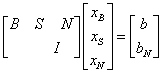where xB , xS , xN called by basics’s variable, superbasics and nonbasics what do accordingly with [B, S, N].

Note: basics’s variable and superbasic is variable one free on bounds.

Theorem following to give that surety nonlinear’s program have solution.

Theorem 3. Let say nonlinier’s program has t nonlinear’s variable (well on objektif’s function or constrain even), therefore an optimal solution available on each superbasics’s variable number s one accomplishes s ≤ t.

Proof. Let is variable non linear regular on appreciative optimal. Problem is rest is linear program for a basic’s solution whatever available (s=0). Its result is trivial if variable nonlinear is regarded as superbasic on early problem. If s=t, available variable nonlinear that current on bounds is nonbasic the so called. Therefore has s ≤ t.

From Theorem 3 secure to mark sense optimal solutions so base 3 get to be made by defenition followings.

Definition 4. Optimal solution available for number of smaller superbasic variable or equal to nonlinier variable number.

Hereafter been given simple algorithm for multi-stage stochastic nonlinear programs.

Set 1. Let K=0, Choose some initial estimates x0, y0 and λ0. Specify a penalty parameter ρ ≥ 0 and convergence tolerance ∈c > 0 .

Set 2. Given xk, yk, λk and ρ , solve the lineraly constrained subproblem (1.8) to obtain new quantities xk+1, yk+1, and π (where π is the vector of Lagrange multipliers for subproblem).

Set 3. Let λK+1 = the first componenets of π.

Set 4. Test convergence (see Definition 5). If optimal, exit.

Set 5. Relinearize the constraints at xk+1.

Set 6. Let K= K+1 and repeat from step 2.

Definition 5. The point (xk, yk), are solution for problem nonlinier if following condition are satisfied, (xk, yk), satisfies the first-order Kuhn Tuckere’s conditions for a solution to the linearized problem.

From the theorems, definitions and algorithm that is given gets to be seen that lagrange projection method can utilized to solve multistage stochastic nonlinear programs.

#### Conclusions

A projected Lagrangian method is a very effective approach for solving medium-size nonlinear programming. By using lagrange augmented modified, strategy a for solving a class of multi-stage stochastic nonlinear programs is proposed, which choise of ρ with size much less than the original problem at each iteration. Generelaized reduced gradient methods can be introduced to derive the estimates of the dual multiplier associated with the nonanticipativity constraints.

#### References

Select your language of interest to view the total content in your interested language

### Article Usage

• Total views: 12177
• [From(publication date):
June-2015 - Aug 23, 2019]
• Breakdown by view type
• HTML page views : 8342
• PDF downloads : 3835

## Post your commentCan't read the image? click here to refresh
###### Peer Reviewed Journals

Make the best use of Scientific Research and information from our 700 + peer reviewed, Open Access Journals

International Conferences 2019-20

Meet Inspiring Speakers and Experts at our 3000+ Global Annual Meetings

Top